diff_months: 11

# 25573 Time Series Econometrics Assignment

Order Code: TV415
• Subject Code :

25573

• University :

University of Technology, Sydney

• Country :

Australia

Question 1

(a) 1 mark, (b)2 marks, (c)2 marks, (d)3 marks

This question is based on the data contained in the Excel file SHARE.XLS. The file contains daily data on the S&P500 from the 2nd of January, 1998 to the 10th of December, 2001 comprising a total of 1027 observations. The S&P500 index is designated PRICE in the file. Generate the series for the percentage log return as:

1. Assume the mean equation for returns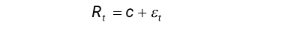is and the variance equation for returns is an ARCH(5). Estimate the model using the first 927 observations. (In EViews, select Object\New Object\Equation. Select                      estimation method ARCH. In the mean equation box that appears, type R followed by C. In the options box, select ARCH 5. In the sample box, type 1 927.)

1. Refer to your notes for the sign restrictions on coefficients that are needed to ensure that the conditional and unconditional variances are positive and finite and that is covariance stationary. Are the sign restrictions for the ARCH specification satisfied in your estimated ARCH equation?
2. The conditional standard deviations are the square roots of the fitted conditional variances from the ARCH estimation. Present a graph of the conditional standard deviation. (After estimating the equation, click View\Conditional SD Graph). Comment.
3. The standardized residuals are the residuals from the mean equation divided by the conditional standard deviations at each period t. Present a histogram and summary statistics of the standardized residuals. Comment. Are these normally distributed? (After estimating the model, click Proc\Make Residual Series. Click for residual type - Standardized. Note the default name for the residual series is resid01. In the series box that appears, click View\Descriptive Statistics\Histogram and Stats

Question 2

There is 3 marks for (a) and 2 mark each for (b) i-iii and  1 mark each for (c) i-iii;

This question is based on the data contained in the Excel file BILLS.xlsx. The file contains monthly data on the yield to maturity on Australian 180-day and 90-day Bank Accepted Bills (denoted bill_180 and bill_90, respectively). The data are from July 1992 to September 2014 and are expressed as percent per annum. Construct a series for the term premium defined as:

term = bill_180 – bill_90

a. Graph the series term. Perform the appropriate Augmented Dickey-Fuller test on the series term. What do you conclude? Make sure you include the null and alternative hypothesis.

b. Estimate the following models for the term premium and briefly comment on your results for each model.  Make sure you make any adjustments as necessarily form your results in part (a)

(i) Model 1: GARCH(1,1)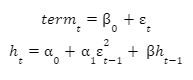(ii) Model 2: GARCH(1,1)-in-Mean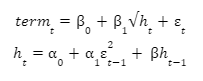(In EViews, select Object\New Object\Equation. Select estimation method ARCH. In the mean equation box that appears, type term followed by c. In the options box, select ARCH 1 and GARCH 1. In the ARCH-M option box, select Std.Dev)

(iii) Model 3: TGARCH(1,1) (with asymmetric adjustment term)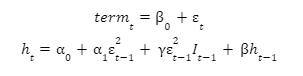(In EViews, select Object\New Object\Equation. Select estimation method ARCH. In the mean equation box that appears, type term followed by c. In the options box, select ARCH 1, GARCH and THRESHOLD ORDER 1)

c. Re-estimate models 1 to 3 by including the lagged term premium in the mean equation. For example, Model 2 is now: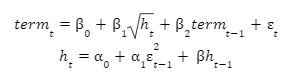Are the results for models 1 to 3 improved by this modification? Justify your answers.

• Uploaded By : Katthy Wills
• Posted on : January 21st, 2023
• Views : 367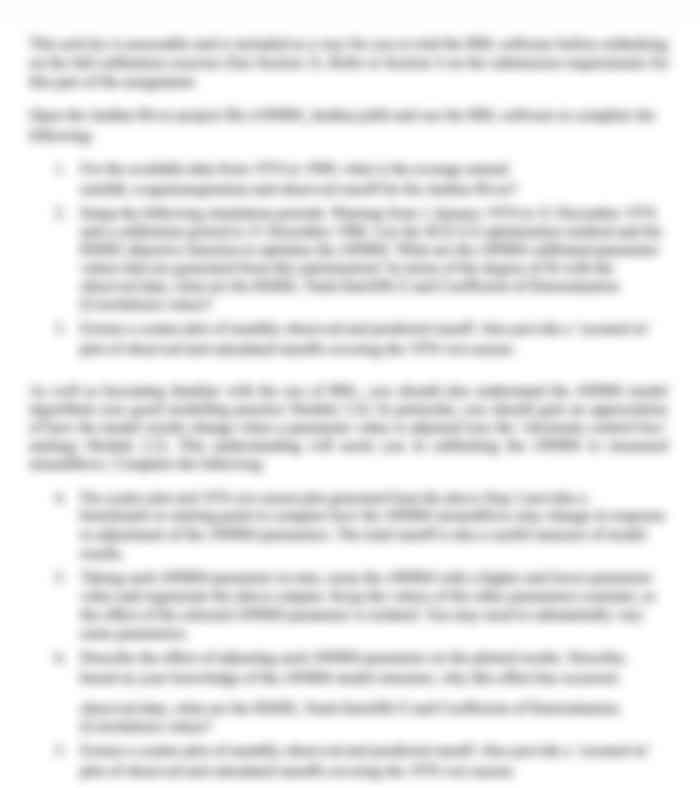## Choose a Plan

80 USD
• All in Gold, plus:
• 30-minute live one-to-one session with an expert
• Understanding Marking Rubric
• Structuring & Formatting
• Referencing & Citing
Most
Popular

### Gold

30 50 USD
• Get the Full Used Solution
(Solution is already submitted and 100% plagiarised.
Can only be used for reference purposes)
Save 33%

### Silver

20 USD
• Journals
• Peer-Reviewed Articles
• Books
• Various other Data Sources – ProQuest, Informit, Scopus, Academic Search Complete, EBSCO, Exerpta Medica Database, and more